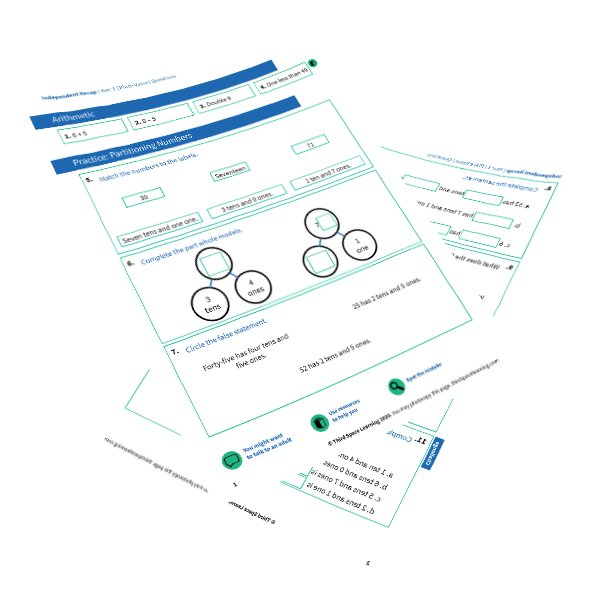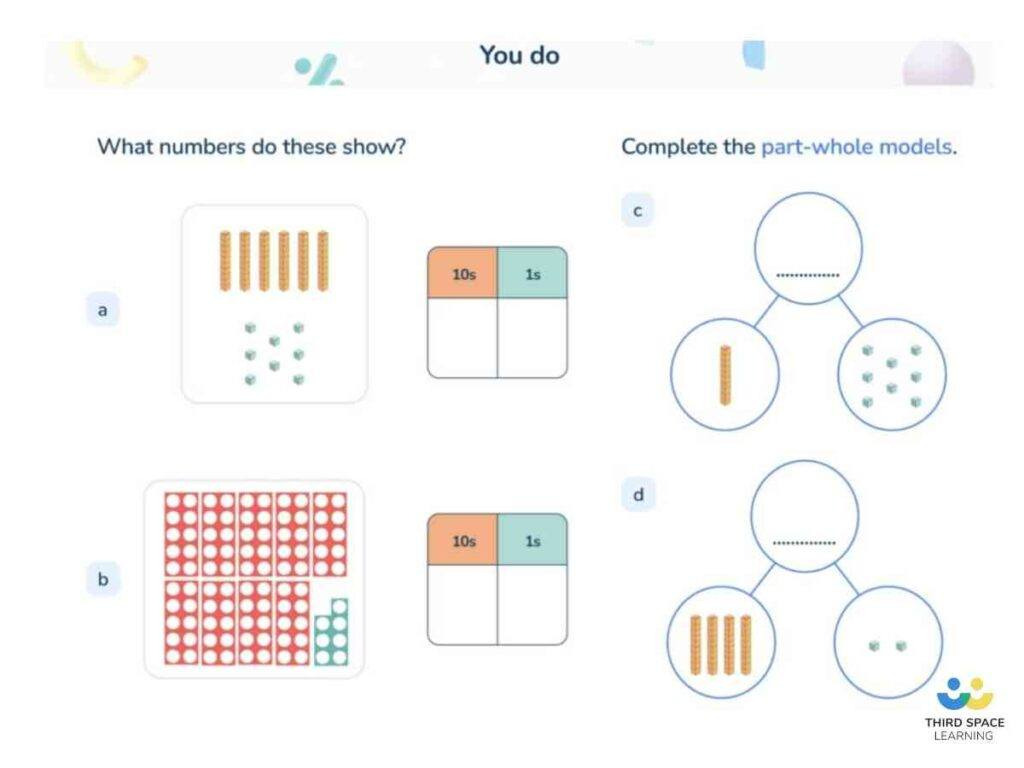Teaching support from the UK’s largest provider of in-school maths tuitionone to one lessonsschools supported

Weekly personalised one to one maths tuition that plugs gaps, builds confidence and boosts progress

See it in action

Hundreds of FREE home learning resources!

Used by thousands of teachers: games, worksheets, daily activities and more!Register for FREE now!
KS2 SATs
KS3 & KS4 Maths
Classroom Teaching
Maths Mastery
Maths Interventions
Home Learning

Weekly personalised one to one maths tuition that plugs gaps, builds confidence and boosts progress

See it in action

# What Is Part Whole Model? Explained For Primary School Parents & Teachers

Here you can find out what the part whole model is, why they are called the ‘part whole model’ and how you can help children to understand the part whole model as part of their maths learning at home.

The part whole model is a way of representing the relationship between a ‘whole’ and its component parts. The visual representation used early on when students learn about the part whole model is known as a cherry diagram (model template shown below), but a bar model and bar modelling can also be used to show the part-whole relationship of numbers.

Read more: What is a bar model

The act of splitting a number into component parts is known as partitioning. The part whole model is a useful model for students to learn and utilise as it will assist with addition and subtraction later on in their mathematical journey. A fraction bar model and bar model multiplication and division wil also be useful.

### What is the part whole model?

The part whole model is a pictorial representation that shows the relationship between a whole and its parts. While usually constructed with just two parts, a whole number can be partitioned into as many component parts as a person may choose. For example, 6 can be partitioned into 2 parts (4 & 2), 3 parts (3, 2 & 1) or even 7 parts (0.5, 0.5, 1, 1, 1, 1, 1).

The greater a student’s understanding of the part-whole diagram, the greater their understanding of the number system and their sense of numberness is. With a greater sense of numberness, the more the students are likely to manipulate numbers for addition and subtraction problem solving and rely less on an algorithm, which may provide them with little understanding of what is actually happening mathematically. An early introduction to gaining this numberness is through the part whole model.FREE Partioning Numbers Worksheet

This time-saving worksheet is for Year 1 partioning numbers and includes 4 arithmetic questions, 9 consolidation questions, and 1 challenge question.Download Free Now!

### Part whole model examples

In the part whole model below, you can see that the whole is 5 and one of the parts is 2. Before learners use abstract numbers, it is important to use objects that can represent cardinality (the amount in a group) as this will be easier for young children to manipulate.

With part whole models, it is important to remember that the parts combine to make the whole. The parts and the whole should not be combined to create a new whole. Rather, the point of the part whole model is to look at what the other missing part would be when combined with the other part that makes the whole. To write this model as a number sentence it would be 2 + __ = 5 or 5 = 2 + __.

A clear use for the part whole model, as demonstrated above, is for students to become familiar with their number bonds to and within certain numbers. The national curriculum for mathematics required students to become fluent in their number bonds to numbers within 20 by the end of year 2. While part whole models will not be the only way that learners become automatic at such procedures, it is one tool that will help them.

Part whole reasoning is being able to understand that whole numbers can be partitioned into any number of component parts. This will then allow the learners to manipulate the order in which numbers may be added or subtracted from each other (what is called the associative law of addition) while still getting to the correct answer. For example, solving 17 + 25 can be done utilising the part whole model is in the following way:

A common use of the part whole model is to partition numbers in accordance with the place value of each digit. This has been demonstrated above. The digit 2 in the tens place has been partitioned to represent 20, and the digit 1 in the tens place has been partitioned to represent 10. These can then be added together to get 30.

The digit 7 which represents the ones can be partitioned into 5 and 2 as this will make adding these to the ones from 25 easier as many learners know the number bond that 5 + 5 is equal to 10. This can be added to the 40 before adding the final 2 to get the answer 42.

While in the initial stages of learning, this can take some time and lead some people to wonder whether it would be better to use a more conventional method, such as column addition or a number line. With time, students will become automatic at the process and there will be no need to write out the models. They will be able to solve such questions more quickly than a person could write out the calculation in a column method.

### What is part part whole?

Depending on the scheme of work used by the schools to deliver their maths curriculum, some may refer to ‘part part whole’. The part part whole model is merely a synonym for the part whole model as discussed above. Personally, I prefer the use of part whole as it describes the relationship that the model attempts to demonstrate. Part part whole narrows students thinking in that there can only be two parts and a whole. This can limit students understanding that a whole can be partitioned into a number of parts.

If you are a premium member of Third Space Learning’s Maths Hub, you can access a CPD video that covers how to teach number bonds using the part-part-whole model.

### When do children learn about the part whole model in school?

The national curriculum does not specify that students must learn this particular model. Nonetheless, many schemes of work do include the part-whole model. It is usually introduced in Reception and continues to be used in KS1.

From my experience, it is used less at KS2 as it is assumed that students can partition numbers mentally. However, this is not always the case and students should feel free to continue using it to support this important skill.One to one lesson from Third Space Learning focusing on part whole model

### How does the part whole model relate to other areas of maths?

As a model, it can be linked to other areas of mathematics where subtraction or addition is required. It is a good stepping stone to build strong mental methods of addition and subtraction as it opens up opportunities for learners to see the associativity law of addition. The part whole model also relates to mental methods where partitioning a number may be useful, such as measure, shape, and addition and subtraction with whole numbers and decimals.

The purpose of these models is to scaffold students’ understanding of numbers. It is therefore unnecessary for learners to actually use these models in the real world. However, the learning and understanding of numbers that students will gain from using the models early on in their mathematics journey will be used when adding in the real world.

For example, when calculating £5 + £7, one could partition 7 into 5 and 2, add the two 5s together to make 10 and then add the 2 to get £12. All that is possible through understanding the part-whole relationship of numbers.

### Part whole model worked examples

1. Partition this number into tens and ones.

We can see that the whole is 89 and the question has asked us to partition this into tens and ones. Those will be the two parts. The 8 in 89 represents the tens, and 8 tens are 80, so that is one part.

The 9 represents 9 ones which can be written as ‘9’. The missing number is 9.

2. Find the whole number.

We can see that the parts of the whole are 50 and 3, so the whole must be the combined total of those numbers. 50 + 3 is 53 so that is my whole.

3. Complete the partition.

The whole is 45 and one part of the whole is 15. We need to find what the remaining part is. By subtracting the known part from the whole, we find the remaining part. 45 less 15 is 30. So the remaining part is 30.

### Part whole model questions

1. Partition 57 into tens and ones.

(Answer: 50 and 7)

2. Find the whole.

3. Complete the partition.

4. Complete the partition.

What is a part whole model example?

For example, when adding 22 and 31, you can partition 22 into 20 and 2, and partition 31 into 30 and 1, then adding together 20 and 30 and then 2 and 1.

What is part whole model for kids?

Whole part model is a way to partition numbers, demonstrating the idea that numbers can be split up into parts.

How do you teach number bonds?

The part whole model is a great method for teaching number bonds.

Wondering about how to explain other key maths vocabulary to your children? Check out our Primary Maths Dictionary, or try these other terms related to part whole model:

Online 1-to-1 maths lessons trusted by schools and teachers
Every week Third Space Learning’s maths specialist tutors support thousands of primary school children with weekly online 1-to-1 lessons and maths interventions. Since 2013 we’ve helped over 125,000 children become more confident, able mathematicians. Learn more or request a personalised quote to speak to us about your needs and how we can help.

Our online tuition for maths programme provides every child with their own professional one to one maths tutor

Related Articles

x#### FREE Partioning Numbers Worksheet

This time-saving worksheet is for Year 1 partioning numbers and includes 4 arithmetic questions, 9 consolidation questions, and 1 challenge question.

#### FREE Partioning Numbers WorksheetThis time-saving worksheet is for Year 1 partioning numbers and includes 4 arithmetic questions, 9 consolidation questions, and 1 challenge question.